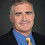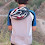## Thursday, June 11, 2015

### A Metric Group Product

A guest post by Dylan McKay, recently graduated from Georgia Tech and soon to be PhD student at Stanford.

[Editor's Note: Turns out the given solution doesn't work and whether a metric group product over the non-negative reals exists remains open. Update 7/10/18: A non-constructive solution was posted on Mathoverflow back in 2010]

Here a cute puzzle motivated by a pair of undergrads and their poor understanding of what the phrase “Algebraic Geometry” really should mean:

Find a function f from the nonnegative reals to the nonnegative reals that satisfies the group axioms and the metric axioms, or prove that there is no such function. That is, find an f:RxR→R such that (R,f) is a group and a metric space. (I am using R to refer to the set of nonnegative real numbers).

As a quick reminder, the group axioms are:

- Closure: f(a,b) must be in R
- Identity: There must exist an element e such that for all a, f(e,a)=f(a,e)=a
- Associative: for all a,b,c, f(f(a,b),c) = f(a,f(b,c))
- Inverse: for all a, there must exist a b such that f(a,b)=e

And the metric axioms are:

- f(a,b) = 0 iff a=b
- f(a,b) <= f(a,c) + f(c,b)
- f(a,b) = f(b,a)

One really bizarre thing about this supposed function is that, what this metric does is essentially guarantee that, given some number x, every other number has a distance from x that is unique -- no other number is exactly that distance away! This is quite counterintuitive to how we think about distance. Each number is its own distance from 0, though, which is very much in line with intuition.

(If you want to solve the puzzle yourself, don't read any further until you do! Unless you want some hints, in which case, look at the next paragraph.)

We can, however, make some other observations that point us in the right direction. For instance, f(e,e) = 0 from the metric axioms and f(e,e) = e from the group axioms, so you get that e = 0. From this and the metric axioms, you get that every element must be it’s own inverse!

Here, we are reminded of a pretty familiar and trusty function, this exclusive-or (XOR) function! And indeed, this will lead us a to a solution.

Here is our candidate function:

Consider x and y in their binary representations. If they do not have the same number of bits to the left of the decimal point (or should it be called the binary point?), pad the one with fewer such bits with 0’s so they have the same number of such bits. Then f(x,y) will be the number represented in binary by the bit-wise exclusive or of these two strings (maintaining the same number of bits to the left of the decimal point, of course). And there we have it: our function!

Now of course, we still need to prove that it satisfies the axioms. But if you do not believe that it does, work it out for yourself! Each axiom is pretty simple to check, especially as we are (for the most part) familiar with this as an extension of an idea for some smaller groups. Well, all of them except our good friend the triangle inequality. This one actually isn’t too bad, but if you have trouble, I will include a hint at the bottom of the post.

Anyway, I hope you liked our puzzle!

Thanks!

-Dylan

Hint for triangle inequality: XOR and addition are really similar, but there is a key difference between them that make a XOR b <= a + b.

1.You mean a function from (R times R) to R, of course.

The next question is whether there are any other examples...

1.Fixed the typo. Thanks.

2.Real numbers can have more than one binary expansion (eg 1.00... is also 0.111...) so you should fix a canonical expansion (this also gives rose to an infinite family of functions f)

3.How do you deal with 0.1111.... (binary)? Is it the same as 1?
What is the result of 0.111.... ^ 1(binary)?
How do you compare the result of (1/3 ^ 2/3) ^ 1 and 1/3 ^ (2/3 ^ 1) (decimal)?

4.Actually a correction on non-uniqueness of representation. It may be problematic.

One can always consider a canonical one. In this representation, digit i is equal to (x << i) & 1.

Each number has one such representation. It doesn't give number that end on 0111.... clearly. If you XOR numbers and don't get a number ending on 01111 .... we are obviously fine

Let's check one corner case when you may get the infinite sequence 01111:

(.0101... XOR a) + (.1010... XOR a) compare with .1111111111 (equal to 1)

as you can see the left part will be also .111111111 in this case. So, the triangle inequality holds.

The other corner case is (a <= 1):
(.0101... XOR .1010...) + (.0101 XOR a) compare with a.

The left part will be at least 1, so, again, the inequality holds.﻿

When I consider a corner case, I consider two specific numbers. However, without a loss of generality, it can be replaced with any pair where the second number is obtained by bit negation of the first number.

5.I talked with Dylan about the comments and the XOR functions doesn't work. Consider f(f(1/3,5/3),f(2/3,1/3)) vs f(f(1/3,f(5/3,2/3)),1/3). With XOR the first case outputs 2 and the second case outputs 1. At this time we don't know whether there is any function that is both a group and a metric over the non-negative reals.

1.I meant the first expression yields 3 and the second case 2.

2.Similarly, and more simply, f(f(1/3,2/3),1)=0 while f(1/3,f(2/3,1))=2 according to the definition of f.

3.Oh, sorry! I just found out that someone had already pointed out exactly the same counter example!

6.I actually like your counter example better, because it is simpler and uses associativity directly.

7.The problem seems to be when the XOR "overflows". Would this work if the XOR was always <= 1?

What if you "smush" [0,Inf) down to [0,1), do the XOR, then expand back to [0,Inf)? E.g., let g(x) = tan^{-1}(x) / (pi/2), and

f(a,b) = g^{-1}(g(a) XOR g(b))

Most things seem to work, but I'm not sure about the triangle inequality. It may involve trig...

Also, as in other peoples' counterexamples, f(g^{-1}(1/3), g^{-1}(2/3)) = f(.111111) = Inf, which is maybe a bit odd, but still OK. I guess f(Inv,Inv) = 0, which makes some sense.

So I'm not sure if this works.

8.Oops, sorry, that should be f(g^{-1}(1/3), g^{-1}(2/3)) = g^{-1}(.111111) = \infty.

Also, if all that the squashing function has to satisfy is that it's strictly increasing, then hyperbolic tangent would also work.

9.If f(a,b) = |a - b| then it satisfies both group axioms and metric axioms.

10.Anonymous 1:21 -- no it doesn't, your f is not associative and so doesn't form a group.

f(f(1,2), 3) = 2 but f(1, f(2, 3)) = 0.

11.previous anonymous: f(a, b) = |a - b| doesn't work since it is not associative. For instance, |1 - |2 - 3|| = 0 but ||1 - 2| - 3| = 2.

12.I think it was pointed out (somewhere) at some point that the "xor" definition of d does work for the nonnegative integers. This extends to the set of rational numbers with denominators a power of 2. The nice thing about this set is that it's very similar to the nonnegative reals wrt addition and the usual order (which are mentioned in the metric axioms). The similarity is the sense that they satisfy a lot of the same first-order properties wrt these operations.

So we have a model of these axioms which also looks an awful lot like the nonnegative reals from a first-order perspective. This suggests looking at an approach to proving the existence of such a metric/group-operation which goes through the compactness theorem of model theory. I am not very familiar with the area, but a friend of mine who is more familiar (but still not an expert) pieced together an argument that seems to work for this; it seems to be mostly standard model theory techniques when applying the compactness theorem. But the basic idea is to include enough statements to ensure that the model resulting from the compactness theorem contains a copy of the nonnegative reals, and then use the existence of the countable model to satisfy the conditions of the compactness theorem. It's possible also that some higher machinery (using Lowenheim-Skolem and the notion of categoricity) might also suffice to prove this; I don't know. But I am fairly convinced that such an object does exist. Consulting a model theorist would yield a more conclusive answer.

13.Please correct me if I am wrong; I have no math degree.

Given that any binary format can be thought of as the integers in base 2 to utilize the XOR function (or any function that work strictly on a binary format) you would have to find a bijection from the reals to the integers.

1.Integers correspond to finite binary strings, whereas the proposed metric uses the representation of real numbers as infinite binary strings

14.Hi. Can you enlighten me regarding this sentence?

>One really bizarre thing about this supposed function is that, what this metric does is essentially guarantee that, given some number x, every other number has a distance from x that is unique -- no other number is exactly that distance away!

Say for example x is 10, both f(11,x) and f(9,x) would give the same value suppose f is the distance function d(a,b) = |a-b|. So can you elaborate on why the metric essentially guarantees that for any x, every other number has a distance from x that is unique? (apparently in this case 11 and 9 has a distance from 10 that is not unique)

Thank you.

1.oh wait. I have misread it. It is existential instead of universal (i.e., "there exists x" rather than "for all x"). Cool. Initially I was really confused at how an Abelian Group with 0 as an identity and satisfy f(a,b) <= f(a,c) + f(c,b) can result in satisfying "for all x every other number has a distance from x that is unique".

Everything makes sense now.

Thanks for the interesting problem by the way. I'll work on it when I'm bored.

15.It seems that this problem has actually been solved! https://mathoverflow.net/questions/16214/is-there-an-associative-metric-on-the-non-negative-reals